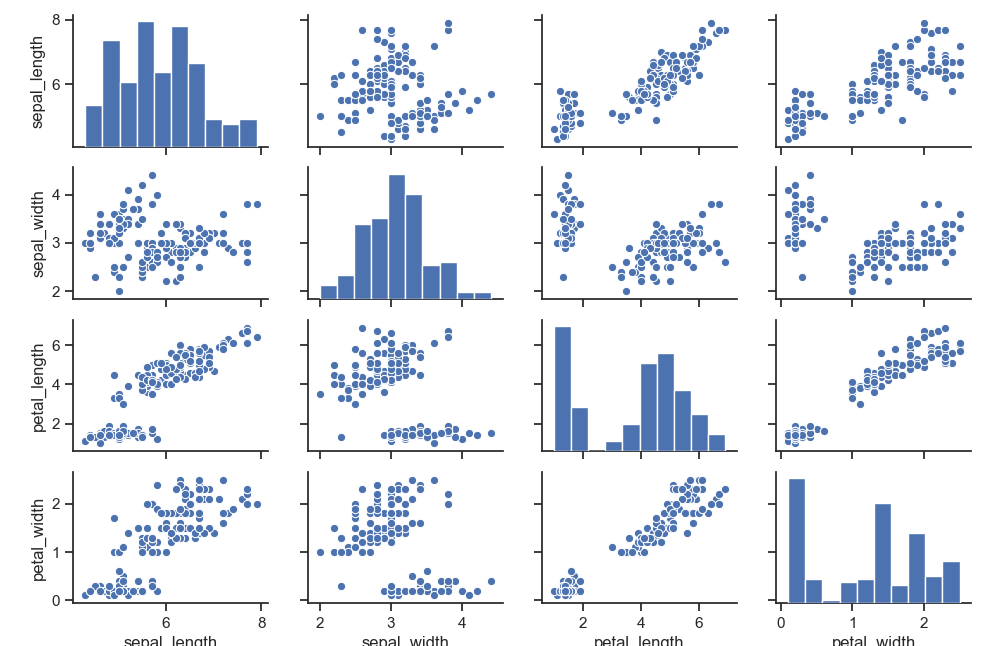A pairplot plot a pairwise relationships in a dataset. The pairplot function creates a grid of Axes such that each variable in data will by shared in the y-axis across a single row and in the x-axis across a single column. That creates plots as shown below.

Related course: Matplotlib Examples and Video Course

## pairplot

### pairplot

The pairplot plot is shown in the image below. Its using the (famous) iris flower data set. The data set has 4 measurements: sepal width, sepal length, petal_length and petal_width. The data contains measurements of different flowers.

This dataset is often used in machine learning, because the measurements and classes (flowers) provide an excellent way to distinguish classes. The data is mapped in the grid below. Because there are 4 measurements, it creates a 4x4 plot.If you prefer a smaller plot, use less variables. For instance, if you only want sepal_width and sepal_length, tha would create a 2x2 plot.

You can change the shape of the distribution.

If you are new to matplotlib, then I highly recommend this course.